#### Value iteration

A simulation-based version of value iteration can be constructed from Q-factors. The reason for their use instead of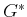is that a minimization over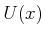will be avoided in the dynamic programming. Avoiding this minimization enables a sample-by-sample approach to estimating the optimal values and ultimately obtaining the optimal plan. The optimal cost-to-go can be obtained from the Q-factors as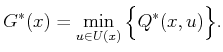(10.96)

This enables the dynamic programming recurrence in (10.46) to be expressed as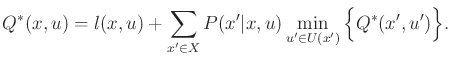(10.97)

By applying (10.97) to the right side of (10.98), it can also be expressed usingas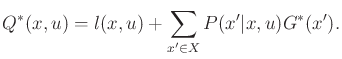(10.98)

If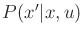and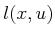were known, then (10.98) would lead to an alternative, storage-intensive way to perform value iteration. After convergence occurs, (10.97) can be used to obtain thevalues. The optimal plan is constructed as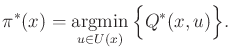(10.99)

Since the costs and transition probabilities are unknown, a simulation-based approach is needed. The stochastic iterative algorithm idea can be applied once again. Recall that (10.96) estimated the cost of a plan by using individual samples and required a convergence-rate parameter,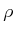. Using the same idea here, a simulation-based version of value iteration can be derived as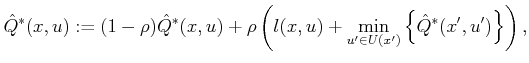(10.100)

in which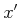is the next state andis the cost obtained from the simulator whenis applied at. Initially, all Q-factors are set to zero. Sample trajectories that arrive at the goal can be generated using simulation, and (10.101) is applied to the resulting states and costs in each stage. Once again, the update equation may appear to be incorrect because the transition probabilities are not explicitly mentioned, but this is taken into account automatically through the simulation.

In most literature, Q-learning is applied to the discounted cost model. This yields a minor variant of (10.101):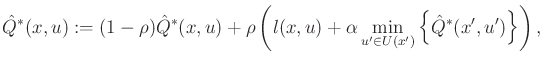(10.101)

in which the discount factor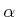appears because the update equation is derived from (10.76).

Steven M LaValle 2020-08-14